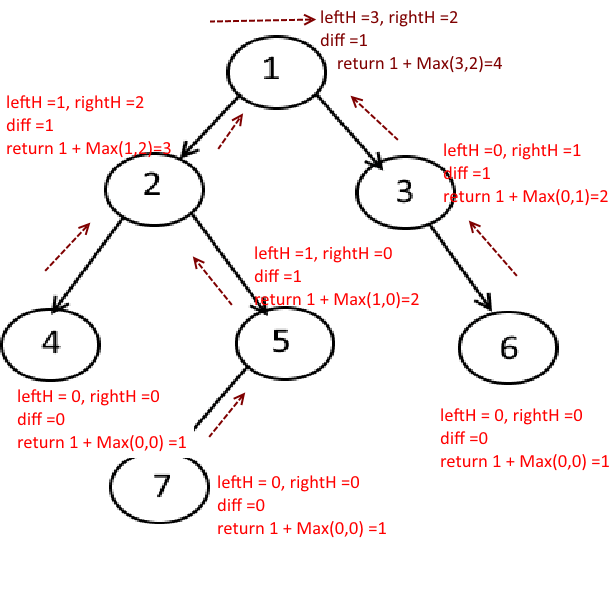Medium

# Find whether a Given Binary Tree is Balanced?

Objective: Given a binary tree, Find whether if a Given Binary Tree is Balanced?

What is a balanced Tree: A balanced tree is a tree in which the difference between the heights of sub-trees of any node in the tree is not greater than one.

Input: A Binary Tree

Output: True and false based on whether the tree is balanced or not.

Example:

Approach :

Naive Approach:
for each node of the tree, get the height of the left subtree and right subtree and check the difference, if it is greater than 1, return false.

Code:

Better Solution:

• Recursion
• Post order traversal technique
• Travel all the way down to leaf nodes and then go up.
• while going up, calculate the left and right subtree height.
• If the difference between them is greater than 1, return -1.
• Else Max(leftHeight, rightHeight) +1 .
• Here you won't actually calculate the height of the subtrees by calling the function, instead, you will store the height at each level and when you go one level up, you add one to it.
• So Time complexity is not O(N^2) but it will be only O(N) but it will have space complexity as O(h) where h is the height of the treeCode:

Output:

`Is Tree Balanced : true Is Tree Balanced : false `